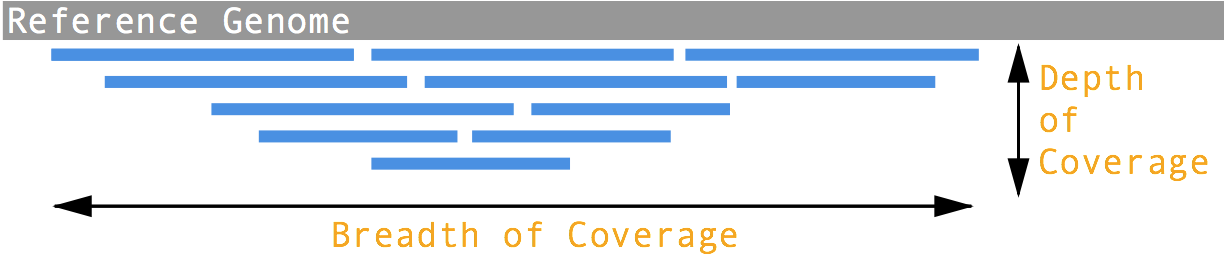### Original Post

What is the difference between depth and coverage in sequencing experiments? Actually – they refer to the same thing, the average number of reads aligned to an individual base. Previously, I had thought coverage referred to the percentage of the genome with aligned reads to it; however the more appropriate term for this is breadth of coverage. This paper more precisely defines what breadth of coverage and depth of coverage mean.If you need to calculate depth of coverage and breadth of coverage you can do so using the python script below. To use the script, feed the function `coverage` a bam file, and the function will return a dictionary of the depth of coverage, breadth of coverage, sum of depths (at every position), and number of bases mapped, for every contig/chromosome individually, and the entire genome as a whole.

Additionally, if you specify the optional second parameter specifying the mitochondrial chromosome, the script will calculate the parameters listed above for the nuclear genome and calculate the ratio of mitochondrial depth of coverage to nuclear depth of coverage. This can act as a proxy for mitochondrial count/content within a cell.

``````#
# This script calculates the depth of coverage and breadth of coverage for a given bam.
# Outputs a dictionary containing the contig/chromosome names and the depth and breadth of coverage for each
# and for the entire genome.
#
# If you optionally specify the name of the mitochondrial chromosome (e.g. mtDNA, chrM, chrMT)
# The script will also generate breadth and depth of coverage for the nuclear genome AND the ratio
# of mtDNA:nuclearDNA; which can act as a proxy in some cases for mitochondrial count within an individual.
#
# Author: Daniel E. Cook
# Website: Danielecook.com
#

import os
import re
from subprocess import Popen, PIPE

def get_contigs(bam):
header, err = Popen(["samtools","view","-H",bam], stdout=PIPE, stderr=PIPE).communicate()
if err != "":
raise Exception(err)
# Extract contigs from header and convert contigs to integers
contigs = {}
for x in re.findall("@SQ\WSN:(?P<chrom>[A-Za-z0-9_]*)\WLN:(?P<length>[0-9]+)", header):
contigs[x] = int(x)
return contigs

def coverage(bam, mtchr = None):
# Check to see if file exists
if os.path.isfile(bam) == False:
raise Exception("Bam file does not exist")
contigs = get_contigs(bam)

# Guess mitochondrial chromosome
mtchr = [x for x in contigs if x.lower().find("m") == 0]
if len(mtchr) != 1:
mtchr = None
else:
mtchr = mtchr

coverage_dict = {}
for c in contigs.keys():
command = "samtools depth -r %s %s | awk '{sum+=\$3;cnt++}END{print cnt \"\t\" sum}'" % (c, bam)
coverage_dict[c] = {}
coverage_dict[c]["Bases Mapped"], coverage_dict[c]["Sum of Depths"] = map(int,Popen(command, stdout=PIPE, shell = True).communicate().strip().split("\t"))
coverage_dict[c]["Breadth of Coverage"] = coverage_dict[c]["Bases Mapped"] / float(contigs[c])
coverage_dict[c]["Depth of Coverage"] = coverage_dict[c]["Sum of Depths"] / float(contigs[c])
coverage_dict[c]["Length"] = int(contigs[c])

# Calculate Genome Wide Breadth of Coverage and Depth of Coverage
genome_length = float(sum(contigs.values()))
coverage_dict["genome"] = {}
coverage_dict["genome"]["Length"] = int(genome_length)
coverage_dict["genome"]["Bases Mapped"] = sum([x["Bases Mapped"] for k, x in coverage_dict.iteritems() if k != "genome"])
coverage_dict["genome"]["Sum of Depths"] = sum([x["Sum of Depths"] for k, x in coverage_dict.iteritems() if k != "genome"])
coverage_dict["genome"]["Breadth of Coverage"] = sum([x["Bases Mapped"] for k, x in coverage_dict.iteritems() if k != "genome"]) / float(genome_length)
coverage_dict["genome"]["Depth of Coverage"] = sum([x["Sum of Depths"] for k, x in coverage_dict.iteritems() if k != "genome"]) / float(genome_length)

if mtchr != None:
# Calculate nuclear breadth of coverage and depth of coverage
ignore_contigs = [mtchr, "genome", "nuclear"]
coverage_dict["nuclear"] = {}
coverage_dict["nuclear"]["Length"] = sum([x["Length"] for k,x in coverage_dict.iteritems() if k not in ignore_contigs ])
coverage_dict["nuclear"]["Bases Mapped"] = sum([x["Bases Mapped"] for k, x in coverage_dict.iteritems() if k not in ignore_contigs])
coverage_dict["nuclear"]["Sum of Depths"] = sum([x["Sum of Depths"] for k, x in coverage_dict.iteritems() if k not in ignore_contigs])
coverage_dict["nuclear"]["Breadth of Coverage"] = sum([x["Bases Mapped"] for k, x in coverage_dict.iteritems() if k not in ignore_contigs]) / float(coverage_dict["nuclear"]["Length"])
coverage_dict["nuclear"]["Depth of Coverage"] = sum([x["Sum of Depths"] for k, x in coverage_dict.iteritems() if k not in ignore_contigs]) / float(coverage_dict["nuclear"]["Length"])

# Calculate the ratio of mtDNA depth to nuclear depth
coverage_dict["genome"]["mt_ratio"] = coverage_dict[mtchr]["Depth of Coverage"] / float(coverage_dict["nuclear"]["Depth of Coverage"])

# Flatten Dictionary
coverage = []
for k,v in coverage_dict.items():
for x in v.items():
coverage += [(k,x, x)]
return coverage``````

Requires BCFTools

### Update: mosdepth (2019-06-21)

If you are looking to calculate coverage, I highly recommend mosdepth.